# GSEB Solutions Class 11 Maths Chapter 15 Statistics Ex 15.1

Gujarat Board GSEB Textbook Solutions Class 11 Maths Chapter 15 Statistics Ex 15.1 Textbook Questions and Answers.

## Gujarat Board Textbook Solutions Class 11 Maths Chapter 15 Statistics Ex 15.1

Find the mean deviation about the mean for the data in questions 1 and 2:
1. 4, 7, 8, 9, 10, 12, 13, 17
2. 38, 70, 48, 40, 42, 55, 63, 46, 54, 44
Solutions to questions 1 and 2:
1. Arithmetic mean $$\bar{x}$$ of 4, 7, 8, 9, 10, 12, 13, 17 is
$$\bar{x}$$ = $$\frac{4+7+8+9+10+12+13+17}{8}$$ = $$\frac{80}{8}$$
= 10.
Σ |xi – $$\bar{x}$$| = 6 + 3 + 2 + 1 + 0 + 2 + 3 + 7
= 24.
= M.D. ($$\bar{x}$$) = $$\frac{\Sigma\left|x_{i}-\bar{x}\right|}{n}$$ = $$\frac{24}{8}$$ = 3.

2. Mean of the given data in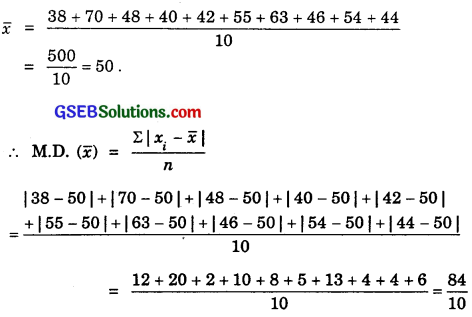= 8.4Find the mean deviation about the mean for the data in questions 3 and 4:
3. 13, 17, 16, 14, 11, 13, 10, 16, 11, 18, 12, 17.
4. 36, 72, 46, 42, 60, 45, 53, 46, 51, 49.
Solutions to questions 3 and 4:
3. The given data is
13, 17, 16, 14, 11, 13, 10, 16, 11, 18, 12, 17.

Arranging it in ascending order:
10, 11, 11, 12, 13, 13, 14, 16, 16, 17, 17, 18.
There are 12 observations.
∴ $$\frac{12}{2}$$ th item = 6th item = 13.
and ($$\frac{12}{2}$$ + 1)th item = 7th item = 14.
∴ Median M = $$\frac{1}{2}$$(13 + 14) = 13.5.
∴ $$\sum_{i=1}^{12}$$|xi – M| = 3.5 + 2.5 + 2.5 + 1.5 + 0.5 + 0.5 + 0.5 + 2.5 + 2.5 + 3.5 + 3.5 + 4.5
= 28.
M.D. (M) = $$\frac{28}{12}$$ = 2.33.

4. The given data is
36, 72, 46, 42, 60, 45, 53, 46, 51, 49.

Arranging the data in ascending order:
36, 42, 45, 46, 46, 49, 51, 53, 60, 72.
The number of observations = 10.
∴ $$\frac{10}{2}$$th = 5th observation = 46.
($$\frac{10}{2}$$ + 1)th = 6th observation = 49.
∴ Median = $$\frac{1}{2}$$(46 + 49) = 47.5.
= |36 – 47.51 + |42 – 47.5| + |45 – 47.5| + |46 – 47.5| + |46 – 47.5| + |49 – 47.5| + |51 – 47.5| + |53 – 47.5| + |60 – 47.5| + |72 – 47.5|
=11.5 + 5.5 + 2.5 + 1.5 + 1.5 + 1.5 + 3.5 + 5.5 + 12.5 + 24.5 = 70.
∴ M.D.(M) = $$\frac{70}{10}$$ = 7.Find the mean deviation about the median for the data in questions 7 to 8: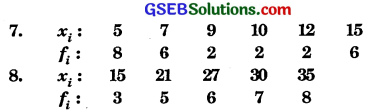Solutions to questions 7 and 8:
7. The table to find cumulative frequency c.f. and median is as follows: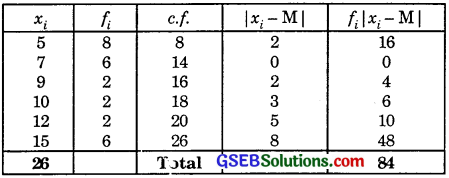Total of the frequencies = 26.
∴Median M = $$\frac{1}{2}$$[$$\frac{26}{2}$$th value + ($$\frac{26}{2}$$ + 1)th value]
= $$\frac{1}{2}$$(13th value + 14th value)
= $$\frac{1}{2}$$(7 + 7) = 7.
∴ Mean deviation from the median
$$\frac{\Sigma f_{i}\left(x_{i}-M\right)}{\Sigma f_{i}}$$ = $$\frac{84}{26}$$ = $$\frac{42}{13}$$ = 3.23.

8. Cumulative frequency distribution of the given data is: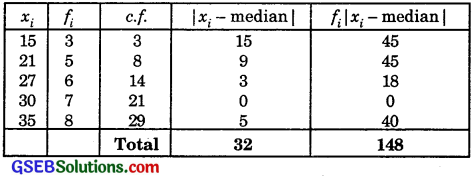Since N = Σfi = 29, which is odd, the median is the ($$\frac{n+1}{2}$$)th observation, i.e., ($$\frac{29+1}{2}$$ = 15)th observation, which is equal to 30. Thus, median is 30.
∴ Mean deviation from the median = $$\frac{\Sigma f_{i}\left|x_{i}-30\right|}{\Sigma f_{i}}$$ = $$\frac{148}{29}$$ = 5.1.

Find the median deviation about mean for the data in questions 9 and 10: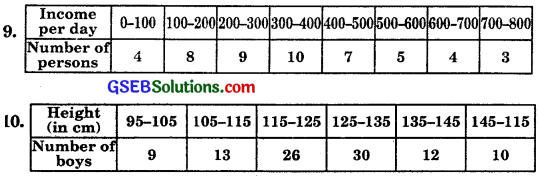Solutions to questions 9 and 10:
9.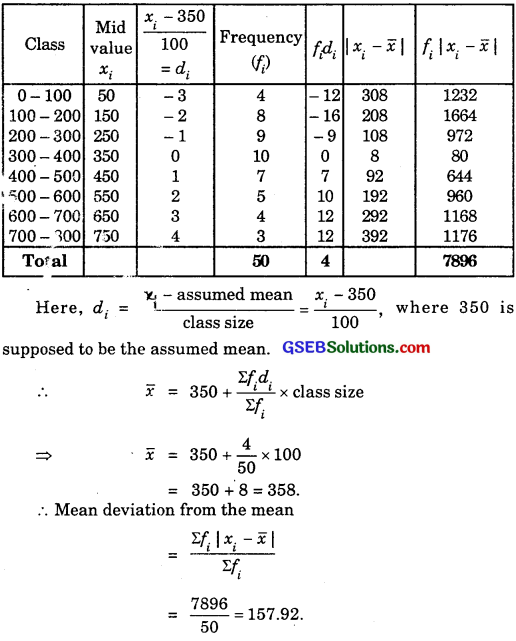10.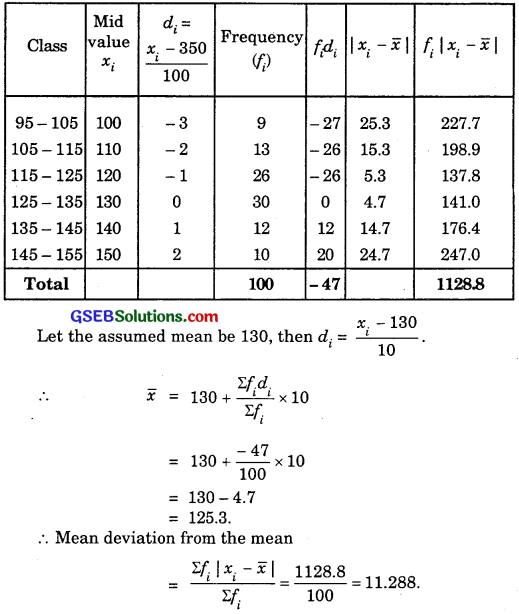Question 11.
Find the mean deviation about median for the following data: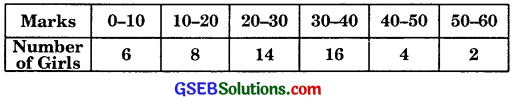Solution:
Table to find cumulative frequencies and Σfi|xi – M| is given below: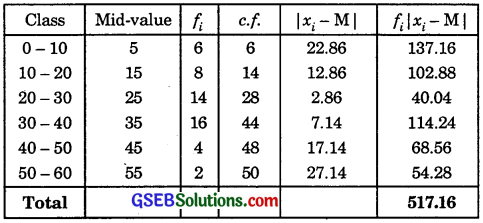Here, $$\frac{N}{2}$$ = $$\frac{50}{2}$$ = 25.
So, median class is 20 – 30
∴ l = 20, f = 14, h = 10.
Median = $$\frac{\frac{N}{2}-C}{f}$$ × h
= 20 + $$\frac{25-14}{14}$$ × 10
= 20 + $$\frac{11}{14}$$ × 10
= 20 + 7.86
= 27.86.
Σfi|xi – M| = 517.16.
= $$\frac{517.16}{50}$$ = 10.34Question 12.
Calculate the mean deviation about median for the age distribution of 100 persons given below: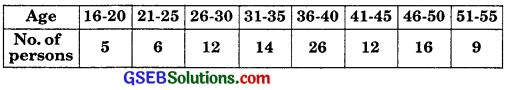Solution:
Continuous frequency distribution, cumulative frequencies and fi|xi – M| are given in the following table: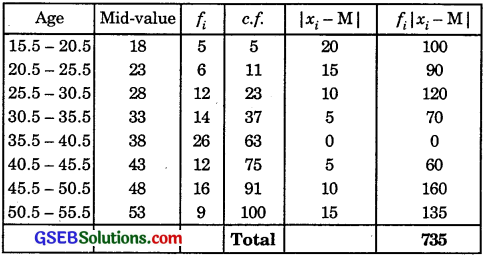Median class 35.5 – 40.5.
∴ l = 35.5 and h = 5, C = 37, f = 26.
∴ Median = l + $$\frac{\frac{N}{2}-C}{f}$$ × h
= 35.5 + $$\frac{50-37}{26}$$ × 5 = 35.5 + $$\frac{13}{26}$$ × 5
= 35.5 + 2.5 = 38.
∴ Σfi|xi – M| = 735..
N = Σfi = 100.
= $$\frac{\Sigma f_{i}\left|x_{i}-M\right|}{N}$$ = $$\frac{735}{100}$$ = 7.35.